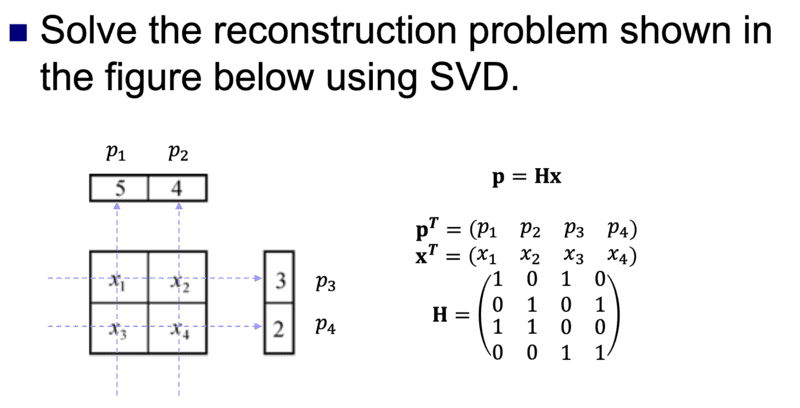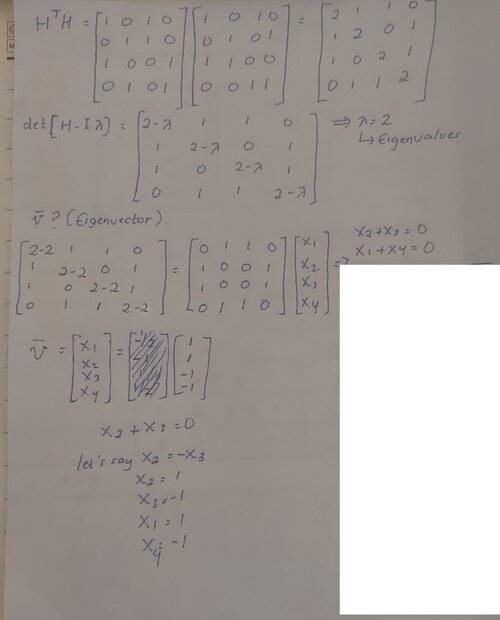# Singular Value Decomposition

nao113
Homework Statement:
Hello, I have question related to SVD. Can anyone give me hints about what should I do to solve this question. I provided the question and my answer on the picture below. I have found the eigenvalues and eigenvector but I m not sure whether it is correct not and then whether this answer already cover the question. Thank you
Relevant Equations:
𝐀=𝐔Σ𝐕∗I am still beginner in this area so it s quite hard for me to understand this one. I am not sure what the output that this question asked me. I thought it might be asked about the value of x1, x2, x3, and x4•Delta2

Staff Emeritus
Homework Helper
Gold Member
Recheck that determinant for ##~\mathbf{H^T H - I} \it \lambda ## .

•Mark44
nao113
Recheck that determinant for ##~\mathbf{H^T H - I} \it \lambda ## .
Hello, thank you the respond, actually, I have correct my mistakes here. I already got the answer from this. But still, can you help me to understand what is U means? and how to get the matrix for it as well as Vt?

#### Attachments

Mentor
But still, can you help me to understand what is U means?
U is a unitary matrix. See https://en.wikipedia.org/wiki/Unitary_matrix.

If U is a complex square matrix, it is unitary if its complex transpose (U*) is also its inverse. I.e., U* U = U U* = I.

Gold Member
Hello, thank you the respond, actually, I have correct my mistakes here. I already got the answer from this. But still, can you help me to understand what is U means? and how to get the matrix for it as well as Vt?
U is one of the parts of the SVD, which is given by U Σ VT.
See: https://en.wikipedia.org/wiki/Singular_value_decomposition
Sometimes the letter S is used in place of Σ, which is the case in the image you've linked to.

The image you linked is trying to show how to calculate the pseudoinverse using the SVD: https://en.wikipedia.org/wiki/Moore–Penrose_inverse. See the heading about 2/3 of the way down the page: "Singular value decomposition (SVD)"

but they have made a mistake. They show the pseudoinverse as: US+VT, but it should be VS+UT

The numeric values they show are correct.

nao113
U is one of the parts of the SVD, which is given by U Σ VT.
See: https://en.wikipedia.org/wiki/Singular_value_decomposition
Sometimes the letter S is used in place of Σ, which is the case in the image you've linked to.

The image you linked is trying to show how to calculate the pseudoinverse using the SVD: https://en.wikipedia.org/wiki/Moore–Penrose_inverse. See the heading about 2/3 of the way down the page: "Singular value decomposition (SVD)"

but they have made a mistake. They show the pseudoinverse as: US+VT, but it should be VS+UT

The numeric values they show are correct.
do you know how to change S to S+?

Gold Member
do you know how to change S to S+?
•